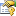﻿ BaseSupportVectorClassification(TModel, TKernel, TInput) PropertiesProperties
NameDescriptionC
Gets or sets the cost values associated with each input vector.Complexity
Complexity (cost) parameter C. Increasing the value of C forces the creation of a more accurate model that may not generalize well. If this value is not set and UseComplexityHeuristic is set to true, the framework will automatically guess a value for C. If this value is manually set to something else, then UseComplexityHeuristic will be automatically disabled and the given value will be used instead.Inputs
Gets or sets the input vectors for training.Kernel
Gets or sets the kernel function use to create a kernel Support Vector Machine. If this property is set, UseKernelEstimation will be set to false.Model
Gets or sets the classifier being learned.
(Inherited from BinaryLearningBaseTModel, TInput.)NegativeWeight
Gets or sets the negative class weight. This should be a value higher than 0 indicating how much of the Complexity parameter C should be applied to instances carrying the negative label.Outputs
Gets or sets the output labels for each training vector.PositiveWeight
Gets or sets the positive class weight. This should be a value higher than 0 indicating how much of the Complexity parameter C should be applied to instances carrying the positive label.Token
Gets or sets a cancellation token that can be used to stop the learning algorithm while it is running.
(Inherited from BinaryLearningBaseTModel, TInput.)UseClassProportions
Gets or sets a value indicating whether the weight ratio to be used between Complexity values for negative and positive instances should be computed automatically from the data proportions. Default is false.UseComplexityHeuristic
Gets or sets a value indicating whether the Complexity parameter C should be computed automatically by employing an heuristic rule. Default is true.UseKernelEstimation
Gets or sets whether initial values for some kernel parameters should be estimated from the data, if possible. Default is true.WeightRatio
Gets or sets the weight ratio between positive and negative class weights. This ratio controls how much of the Complexity parameter C should be applied to the positive class.
TopSee Also